# Class 6 Maths NCERT Solutions for Chapter 13 Symmetry Ex – 13.1

## Symmetry

Question 1.
List any four symmetrical objects from your home or school.

Solution:
List of four symmetrical objects from home or school are
(i) An electric tube
(ii) A glass
(iii) An electric bulb
(iv) A fan

Question 2.
For the given figure, which one is the mirror line, l1 or l2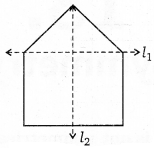Solution:
From the given figure, clearly l2 is the mirror line.

Question 3.
Identify the shapes given below. Check whether they are symmetric or not. Draw the line of symmetry as well.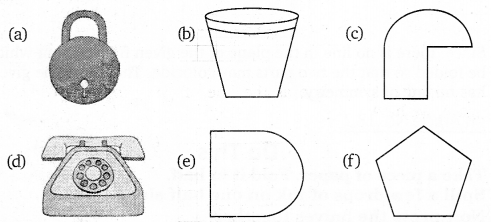Solution:
Figures (a), (b), (d), (e) and (f) are symmetrical and their line of symmetry is shown as a dotted line.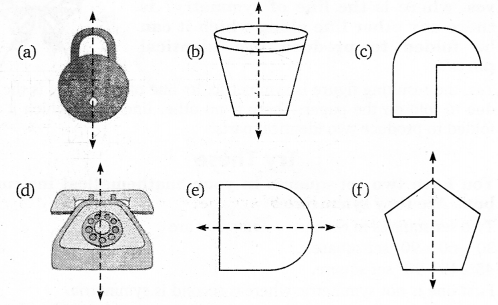Question 4.
Copy the following on a squared paper. A square paper is what you would have used in your arithmetic notebook in earlier classes. Then complete them such that the dotted line is the line of symmetry.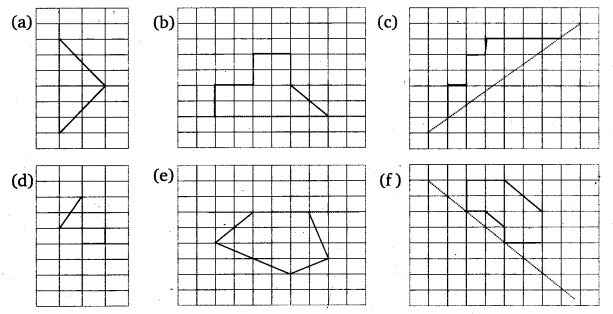Solution:
Keeping the dotted line as the line of symmetry and completing the figure, we haveQuestion 5.
In the figure, l is the line of symmetry. Complete the diagram to make it symmetric.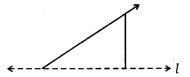Solution:
Complete diagram is as under:Question 6.
In the figure, l is the line of symmetry. Draw the image of the triangle and complete the diagram so that it becomes symmetric.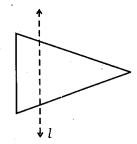Solution:
The image of the triangle is shown such that the complete diagram becomes symmetric.